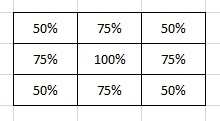BrainDen.com - Brain Teasers
• 0

# Probability of picking a point in a divided rectangle

## Question

Pretend you have a rectangle that is divided into two smaller rectangles where the area of one rectangle is twice that of the other rectangle.  If you were to pick a point at random inside the rectangle, what is the probability that the point is within the larger rectangle?

## Recommended Posts

• 0
Spoiler

According to probability theory for finite sets, the probability P(larger) = Size(larger)/(Size(larger)+Size(smaller)). That would suggest the answer to the OP is 1/2 owing to the fact that the points within the smaller and larger rectangles have the same cardinality, namely the cardinality of the continuum, or Aleph1.

But the formula does not hold for infinite sets, nor do cardinalities of infinite sets behave like ordinary numbers.

The answer to the OP is 2/3, which can be seen as follows: draw a uniform square grid across the containing rectangle. Now ask: If you were to pick a point at random within the containing rectangle, what is the probability that the point will be inside a square belonging to the larger of the two sub-rectangles? Since, no matter how fine we make the grid, the number of squares is finite, and the number of squares in the larger rectangle is twice that of the smaller, we can use P(larger) = 2n/(n + 2n) = 2/3, where n is the number of grid squares in the smaller rectangle. That value is unchanged in the limit n -> infinity

##### Share on other sites

• 0
Spoiler

SpoilerEdited by TimeSpaceLightForce
##### Share on other sites

• 0

That is right.. with 4 partition lines occurring 25% of the time.

Spoiler

(4X50%+4X75%+100%)/9=66.67%

Edited by TimeSpaceLightForce

## Join the conversation

You can post now and register later. If you have an account, sign in now to post with your account.×   Pasted as rich text.   Paste as plain text instead

Only 75 emoji are allowed.

×   Your previous content has been restored.   Clear editor

×   You cannot paste images directly. Upload or insert images from URL.